# 4. Transfer Learning

• new data set is small, new data is similar to original training data
• new data set is small, new data is different from original training data
• new data set is large, new data is similar to original training data
• new data set is large, new data is different from original training data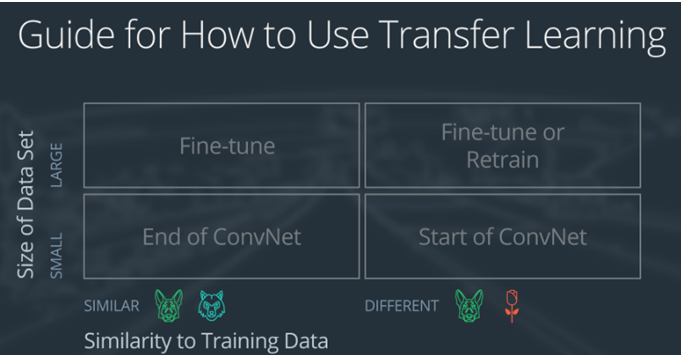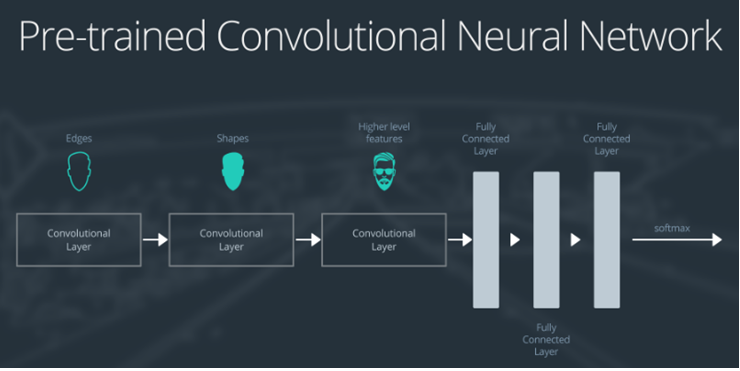1. 第一层卷积检测出图片边界
2. 第二层卷积检测出形状
3. 第三层卷积检测出更高层次的特征

## Case 1: Small Data Set, Similar Data

• slice off(切开) the end of the neural network
• add a new fully connected layer that matches the number of classes in the new data set
• randomize the weights of the new fully connected layer; freeze all the weights from the pre-trained network
• train the network to update the weights of the new fully connected layer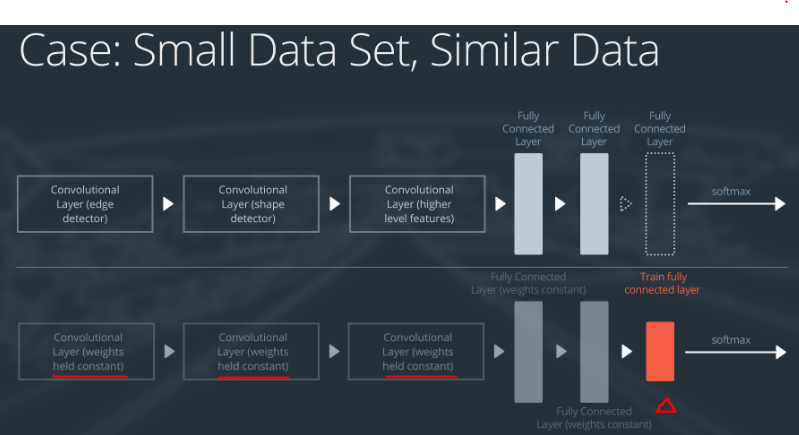## Case 2: Small Data Set, Different Data

• slice off most of the pre-trained layers near the beginning of the network
• add to the remaining pre-trained layers a new fully connected layer that matches the number of classes in the new data set
• randomize the weights of the new fully connected layer; freeze all the weights from the pre-trained network
• train the network to update the weights of the new fully connected layer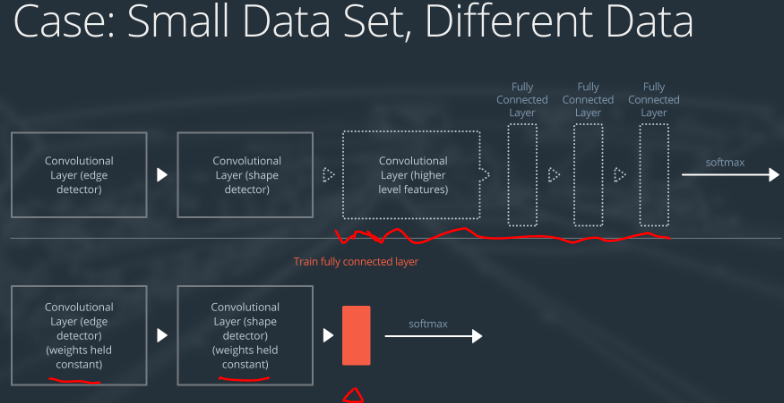## Case 3: Large Data Set, Similar Data

• remove the last fully connected layer and replace with a layer matching the number of classes in the new data set
• randomly initialize the weights in the new fully connected layer
• initialize the rest of the weights using the pre-trained weights
• re-train the entire neural network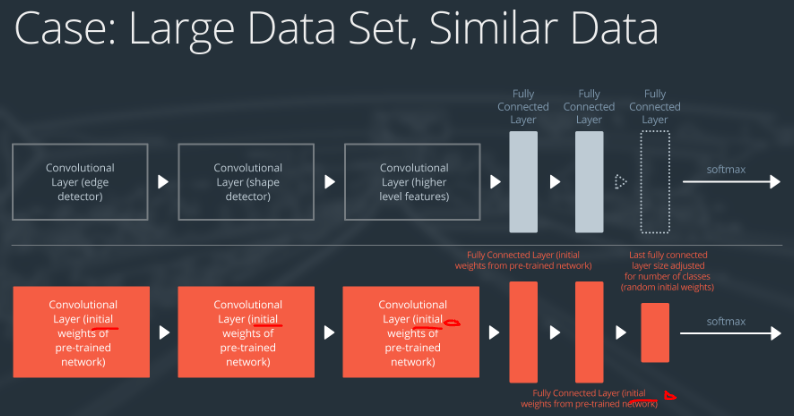## Case 4: Large Data Set, Different Data

• remove the last fully connected layer and replace with a layer matching the number of classes in the new data set
• retrain the network from scratch with randomly initialized weights
• alternatively, you could just use the same strategy as the “large and similar” data case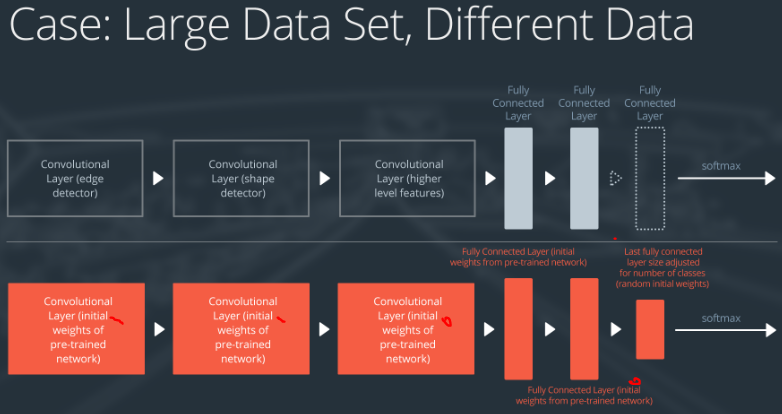# 7. AlexNet

1. 利用GPU并行运算的特性，加速了神经网络的训练
2. 使用ReLU作为激活函数修正线性单元
3. 使用Dropout防止过拟合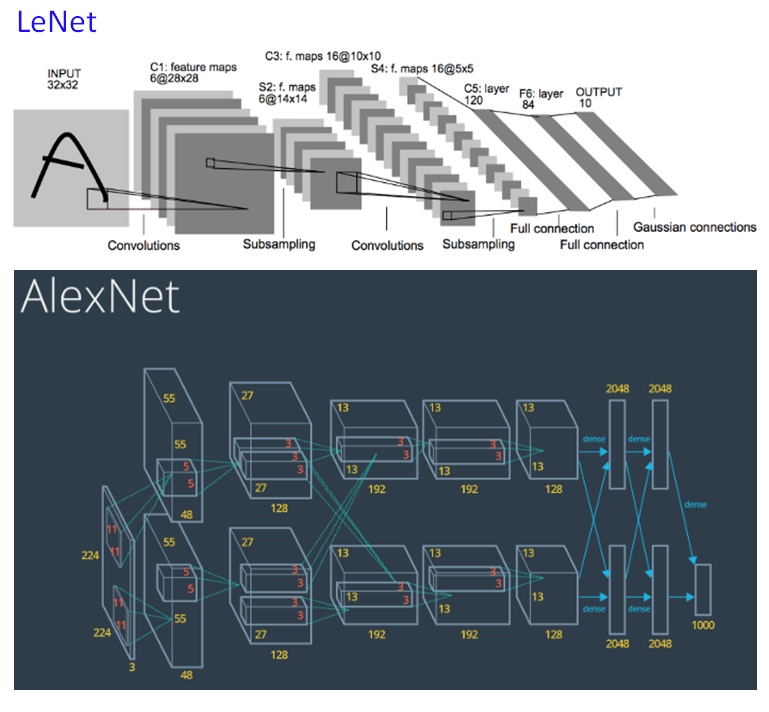# 9. VGG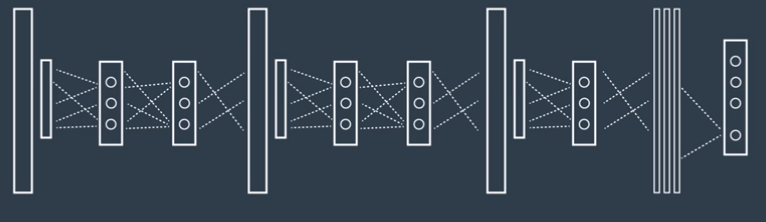VGG in Keras:

Keras中有两个版本的VGG，分别是VGG16和VGG19，对应的layer数量不同。

``````from keras.applications.vgg16 import VGG16

model = VGG16(weights='imagenet', include_top=False)
``````

• 表示加载imagenet的权重参数
• include_top为false表示不需要使用神经网络最后的一层，在imagenet中有1000个label，一般不需要那么多，所以这里设置false

``````from keras.preprocessing import image
from keras.applications.vgg16 import preprocess_input
import numpy as np

img_path = 'your_image.jpg'
x = image.img_to_array(img)
x = np.expand_dims(x, axis=0)
x = preprocess_input(x)
``````

## 1. Demo: VGG without Pre-trained Weights

Load example images and pre-process them

``````# Load our images first, and we'll check what we have
from glob import glob
import matplotlib.image as mpimg
import matplotlib.pyplot as plt
from keras.preprocessing import image
from keras.applications.vgg16 import preprocess_input
import numpy as np

image_paths = glob('images/*.jpg')

i = 2 # Can change this to your desired image to test
img_path = image_paths[i]
x = image.img_to_array(img)
x = np.expand_dims(x, axis=0)
x = preprocess_input(x)
``````

Load VGG16 model, but without pre-trained weights

This time, we won’t use the pre-trained weights, so we’ll likely get so wacky predictions.

``````# Note - this will likely need to download a new version of VGG16
from keras.applications.vgg16 import VGG16, decode_predictions

# Load VGG16 without pre-trained weights
model = VGG16(weights=None)

# Perform inference on our pre-processed image
predictions = model.predict(x)

# Check the top 3 predictions of the model
print('Predicted:', decode_predictions(predictions, top=3))
``````

## 2. Demo: Using VGG with Keras

``````# Load our images first, and we'll check what we have
from glob import glob
import matplotlib.image as mpimg
import matplotlib.pyplot as plt

image_paths = glob('images/*.jpg')

# Print out the image paths
print(image_paths)

# View an example of an image
plt.imshow(example)
plt.show()
``````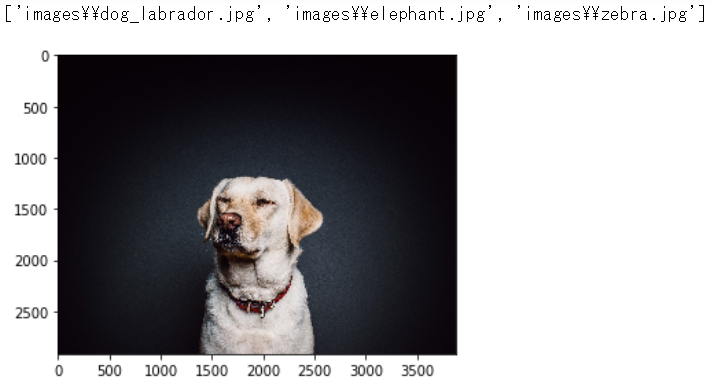Pre-process an image

Note that the image.load_img() function will re-size our image to 224x224 as desired for input into this VGG16 model, so the images themselves don’t have to be 224x224 to start.

``````# Here, we'll load an image and pre-process it
from keras.preprocessing import image
from keras.applications.vgg16 import preprocess_input
import numpy as np

i = 0 # Can change this to your desired image to test
img_path = image_paths[i]
x = image.img_to_array(img)
x = np.expand_dims(x, axis=0)
x = preprocess_input(x)
``````

We won’t throw out the top fully-connected layer this time when we load the model, as we actually want the true ImageNet-related output. However, you’ll learn how to do this in a later lab. The inference will be a little slower than you might expect here as we are not using GPU just yet.

Note also the use of `decode_predictions` which will map the prediction to the class name.

``````# Note - this will likely need to download a new version of VGG16
from keras.applications.vgg16 import VGG16, decode_predictions

model = VGG16(weights='imagenet')

# Perform inference on our pre-processed image
predictions = model.predict(x)

# Check the top 3 predictions of the model
print('Predicted:', decode_predictions(predictions, top=3))
``````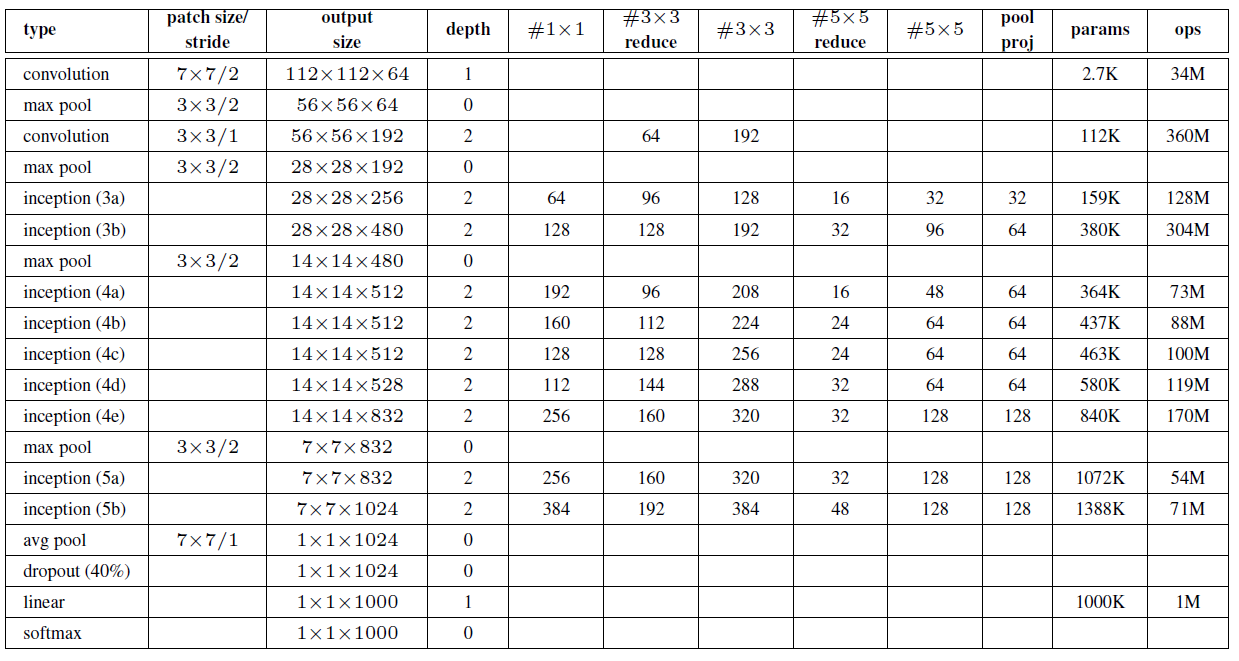``````from keras.applications.inception_v3 import InceptionV3

model = InceptionV3(weights='imagenet', include_top=False)
``````

# 12. ResNet

Imagenet2015年微软使用的网络，将图片的识别错误率降低到了3%，其主要特点是高达152层的网络。对比下层数：

ResNet 152
AlexNet 8
VGG 19

``````from keras.applications.resnet50 import ResNet50

model = ResNet50(weights='imagenet', include_top=False)
``````

# 13. Without Pre-trained Weights

## 1. Demo: VGG without Pre-trained Weights

Load example images and pre-process them

``````# Load our images first, and we'll check what we have
from glob import glob
import matplotlib.image as mpimg
import matplotlib.pyplot as plt
from keras.preprocessing import image
from keras.applications.vgg16 import preprocess_input
import numpy as np

image_paths = glob('images/*.jpg')

i = 2 # Can change this to your desired image to test
img_path = image_paths[i]
x = image.img_to_array(img)
x = np.expand_dims(x, axis=0)
x = preprocess_input(x)
``````

Load VGG16 model, but without pre-trained weights

This time, we won’t use the pre-trained weights, so we’ll likely get so wacky predictions.

``````# Note - this will likely need to download a new version of VGG16
from keras.applications.vgg16 import VGG16, decode_predictions

# Load VGG16 without pre-trained weights
model = VGG16(weights=None)

# Perform inference on our pre-processed image
predictions = model.predict(x)

# Check the top 3 predictions of the model
print('Predicted:', decode_predictions(predictions, top=3))
``````

## 2. Demo: Using VGG with Keras

``````# Load our images first, and we'll check what we have
from glob import glob
import matplotlib.image as mpimg
import matplotlib.pyplot as plt

image_paths = glob('images/*.jpg')

# Print out the image paths
print(image_paths)

# View an example of an image
plt.imshow(example)
plt.show()
``````Pre-process an image

Note that the image.load_img() function will re-size our image to 224x224 as desired for input into this VGG16 model, so the images themselves don’t have to be 224x224 to start.

``````# Here, we'll load an image and pre-process it
from keras.preprocessing import image
from keras.applications.vgg16 import preprocess_input
import numpy as np

i = 0 # Can change this to your desired image to test
img_path = image_paths[i]
x = image.img_to_array(img)
x = np.expand_dims(x, axis=0)
x = preprocess_input(x)
``````

We won’t throw out the top fully-connected layer this time when we load the model, as we actually want the true ImageNet-related output. However, you’ll learn how to do this in a later lab. The inference will be a little slower than you might expect here as we are not using GPU just yet.

Note also the use of `decode_predictions` which will map the prediction to the class name.

``````# Note - this will likely need to download a new version of VGG16
from keras.applications.vgg16 import VGG16, decode_predictions

model = VGG16(weights='imagenet')

# Perform inference on our pre-processed image
predictions = model.predict(x)

# Check the top 3 predictions of the model
print('Predicted:', decode_predictions(predictions, top=3))
``````

# 14. Lab: Transfer Learning

1. 添加新的网络层
2. 冻结权重

## 14.1 GPU usage

``````# Set a couple flags for training - you can ignore these for now
freeze_flag = True  # `True` to freeze layers, `False` for full training
weights_flag = 'imagenet' # 'imagenet' or None
preprocess_flag = True # Should be true for ImageNet pre-trained typically

from keras.applications.inception_v3 import InceptionV3

# We can use smaller than the default 299x299x3 input for InceptionV3
# which will speed up training. Keras v2.0.9 supports down to 139x139x3
input_size = 139

# Using Inception with ImageNet pre-trained weights
inception = InceptionV3(weights=weights_flag, include_top=False,
input_shape=(input_size,input_size,3))
``````

## 14.2 Pre-trained with frozen weights

You can freeze layers by setting `layer.trainable` to False for a given `layer`. Within a `model`, you can get the list of layers with `model.layers`.

``````if freeze_flag == True:
## TODO: Iterate through the layers of the Inception model
##       loaded above and set all of them to have trainable = False
for layer in inception.layers:
layer.trainable = False
``````

## 14.3 Dropping layers

You can drop layers from a model with `model.layers.pop()`. Before you do this, you should check out what the actual layers of the model are with Keras’s `.summary()` function.

``````## TODO: Use the model summary function to see all layers in the
inception.summary()
``````

``````from keras.layers import Input, Lambda
import tensorflow as tf

# Makes the input placeholder layer 32x32x3 for CIFAR-10
cifar_input = Input(shape=(32,32,3))

# Re-sizes the input with Kera's Lambda layer & attach to cifar_input
resized_input = Lambda(lambda image: tf.image.resize_images(
image, (input_size, input_size)))(cifar_input)

# Feeds the re-sized input into Inception model
# You will need to update the model name if you changed it earlier!
inp = inception(resized_input)
``````

``````# Imports fully-connected "Dense" layers & Global Average Pooling
from keras.layers import Dense, GlobalAveragePooling2D

## TODO: Setting `include_top` to False earlier also removed the
##       GlobalAveragePooling2D layer, but we still want it.
##       Add it here, and make sure to connect it to the end of Inception
x = GlobalAveragePooling2D()(inp)

## TODO: Create two new fully-connected layers using the Model API
##       format discussed above. The first layer should use `out`
##       as its input, along with ReLU activation. You can choose
##       how many nodes it has, although 512 or less is a good idea.
##       The second layer should take this first layer as input, and
##       be named "predictions", with Softmax activation and
##       10 nodes, as we'll be using the CIFAR10 dataset.
x = Dense(512, activation = 'relu')(x)
predictions = Dense(10, activation = 'softmax')(x)
``````
``````# Imports the Model API
from keras.models import Model

# Creates the model, assuming your final layer is named "predictions"
model = Model(inputs=cifar_input, outputs=predictions)

# Compile the model

# Check the summary of this new model to confirm the architecture
model.summary()
``````

``````Layer (type)                 Output Shape              Param #
=================================================================
input_2 (InputLayer)         (None, 32, 32, 3)         0
_________________________________________________________________
lambda_1 (Lambda)            (None, 139, 139, 3)       0
_________________________________________________________________
inception_v3 (Model)         (None, 3, 3, 2048)        21802784
_________________________________________________________________
global_average_pooling2d_1 ( (None, 2048)              0
_________________________________________________________________
dense_1 (Dense)              (None, 512)               1049088
_________________________________________________________________
dense_2 (Dense)              (None, 10)                5130
=================================================================
Total params: 22,857,002
Trainable params: 1,054,218
Non-trainable params: 21,802,784
_________________________________________________________________
``````

## 14.5 GPU time

The rest of the notebook will give you the code for training, so you can turn on the GPU at this point - but first, make sure to save your jupyter notebook. Once the GPU is turned on, it will load whatever your last notebook checkpoint is.

While we suggest reading through the code below to make sure you understand it, you can otherwise go ahead and select Cell > Run All (or Kernel > Restart & Run All if already using GPU) to run through all cells in the notebook.

``````from sklearn.utils import shuffle
from sklearn.preprocessing import LabelBinarizer
from keras.datasets import cifar10

(X_train, y_train), (X_val, y_val) = cifar10.load_data()

# One-hot encode the labels
label_binarizer = LabelBinarizer()
y_one_hot_train = label_binarizer.fit_transform(y_train)
y_one_hot_val = label_binarizer.fit_transform(y_val)

# Shuffle the training & test data
X_train, y_one_hot_train = shuffle(X_train, y_one_hot_train)
X_val, y_one_hot_val = shuffle(X_val, y_one_hot_val)

# We are only going to use the first 10,000 images for speed reasons
# And only the first 2,000 images from the test set
X_train = X_train[:10000]
y_one_hot_train = y_one_hot_train[:10000]
X_val = X_val[:2000]
y_one_hot_val = y_one_hot_val[:2000]
``````
``````# Use a generator to pre-process our images for ImageNet
from keras.preprocessing.image import ImageDataGenerator
from keras.applications.inception_v3 import preprocess_input

if preprocess_flag == True:
datagen = ImageDataGenerator(preprocessing_function=preprocess_input)
val_datagen = ImageDataGenerator(preprocessing_function=preprocess_input)
else:
datagen = ImageDataGenerator()
val_datagen = ImageDataGenerator()
``````
``````# Train the model
batch_size = 32
epochs = 5
# Note: we aren't using callbacks here since we only are using 5 epochs to conserve GPU time
model.fit_generator(datagen.flow(X_train, y_one_hot_train, batch_size=batch_size),
steps_per_epoch=len(X_train)/batch_size, epochs=epochs, verbose=1,
validation_data=val_datagen.flow(X_val, y_one_hot_val, batch_size=batch_size),
validation_steps=len(X_val)/batch_size)
``````

## 14.6 Test without frozen weights, or by training from scratch

Training Mode Val Acc @ 1 epoch Val Acc @ 5 epoch Time per epoch
Frozen weights 65.5% 70.3% 50 seconds
Unfrozen weights 50.6% 71.6% 142 seconds
No pre-trained weights 19.2% 39.2% 142 seconds